# Game Of Prediction

A game of prediction is a triple, where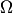is an outcome space,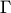is a decision space, and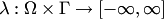is the loss function. Important special cases are those of:

• point predictions, where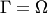;
• probability predictions, whereis the set of all probability measures on; ifis finite, the usual loss function is the log loss;
• region predictions, whereis the set of all subsets of; the usual loss function is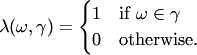The case of region predictions (in particular, interval predictions) is the main object of study in conformal prediction but has never been studied in competitive on-line prediction. This is stated as open problem in the article competitive on-line interval prediction.